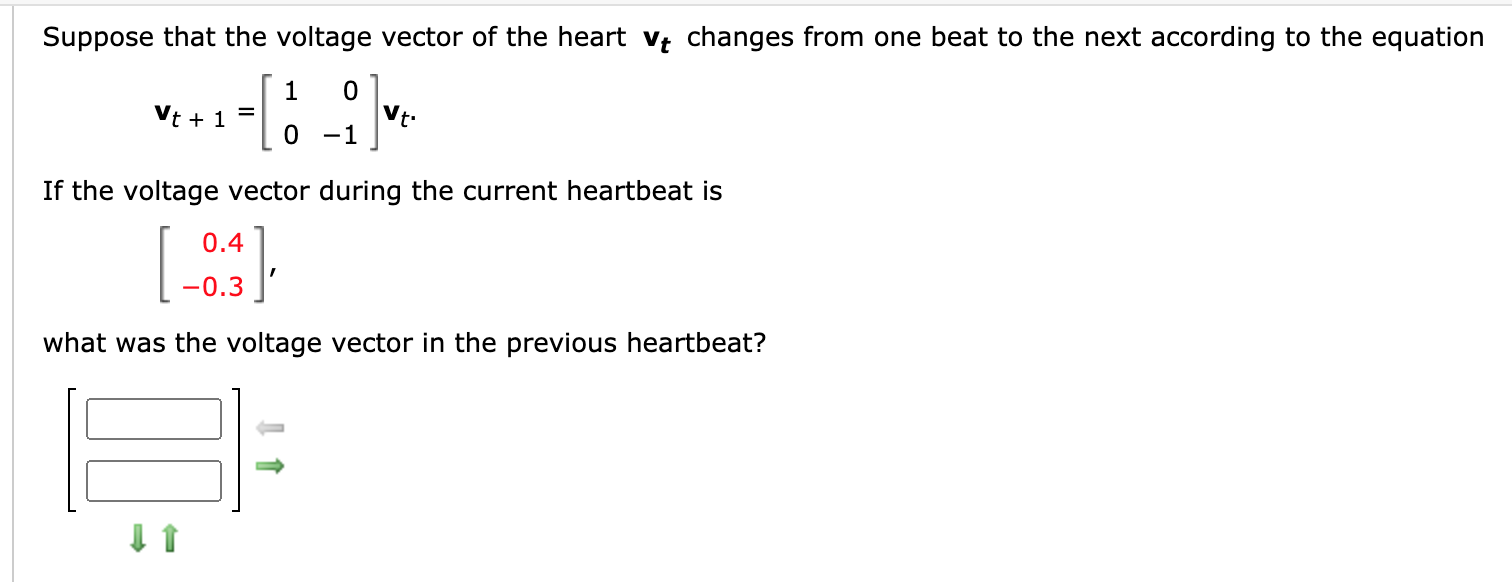# Question Solved1 AnswerSuppose that the voltage vector of the heart Vt changes from one beat to the next according to the equation Vt + 1 --[6 -2] Vt: If the voltage vector during the current heartbeat is 0.4 0.3 what was the voltage vector in the previous heartbeat?Transcribed Image Text: Suppose that the voltage vector of the heart Vt changes from one beat to the next according to the equation Vt + 1 --[6 -2] Vt: If the voltage vector during the current heartbeat is 0.4 0.3 what was the voltage vector in the previous heartbeat?
More
Transcribed Image Text: Suppose that the voltage vector of the heart Vt changes from one beat to the next according to the equation Vt + 1 --[6 -2] Vt: If the voltage vector during the current heartbeat is 0.4 0.3 what was the voltage vector in the previous heartbeat?